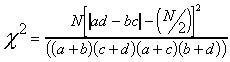Chi square

NOMINAL DATA: Inference

A. Chi square (for 2 x 2 tables):a b a+b c d c+d a+c b+d N

Assumptions:

1) independent variables

2) every fe > 5

3) requires a large sample of 100 or more if not normally distributed

also: d.f. = (R - 1)(C - 1)

where: R = number of rows & C = number of columns

Example:

 MALE FEMALE YES 22 41 63 NO 19 18 37 41 59 100

Does sexually explicit materials lead to rape?

Q: Can this association be generalized to the whole population?

A: 100(|22(18) - 19(41)|- 100/2 )2 = 11,088,900 = 1.967
----(63)(37)(41)(59) -----------------5,638,689

Interpretation: Use table (see appendix).

Portion of a Chi square table:
d.f. p =.05 p = .01

1 ------3.84 ----6.64

2 ------5.99 ----9.21

3------ : --------:

Compute degrees of freedom

Example (above): d.f.= (R - 1)(C - 1)

= (2 - 1)(2 - 1)

= 1(1) = 1

Look on table: with d.f. = 1, if 2 = 3.84, p = .05.

If 2 = 6.64, p = .01. The example 2 = 1.97

Imagine that the table is a continuum:

p > .05 p = .05 p < .05 p = .01 p < .01

0 -1.97----3.84-------------- 6.64

can't can generalize

So the answer to the question: No, one cannot generalize because p >.05 (which means that the chances of being wrong is greater than 5/100).

Size of the sample affects the outcome, greater the size, greater the inferential power of the analysis. If the sample had been quadrupled in size, what would have been the outcome?

Example: 400(|88(72) - 76(164)| - 400/2)2 = 9.74
--------------(164)(236)(148)(252)

Now, the chances of being wrong is less than 1/100. One can generalize to the whole population.

B. Chi square for any size table:= [(fo - fe)2] / fe

Where: fo = number in a cell

fe = (row total)(column total)
------------------N

Assumptions: 1) independent variable 2) every fe > 5

Also: d.f. = (R - 1)(C - 1)

Example:

 Type of Marriage Divorced Still Married homogamous 193 245 438 hypergamous 92 108 200 hypogamous 122 96 218 407 449 856

Q: Is this sample association inferable to the whole population?

A: Make a table to compute data:

 fo fe fe fo - fe (fo - fe)2 [(fo - fe)2] / fe 193 [(438)(407)]/856 208.3 -15.3 234.1 1.12 92 [(200)(407)]/856 95.1 -3.1 9.6 .10 122 [(218)(407)]/856 103.7 18.3 334.9 3.23 245 [(438)(449)]/856 229.7 15.3 234.1 1.02 108 [(200)(449)]/856 104.9 3.1 9.6 .09 96 [(218)(449)]/856 114.3 -18.3 334.9 2.93 856* 0* 8.49

*These totals must = N and 0, respectively, or a math error was made!.= 8.49; but it can't be used as it is.

Must know degrees of freedom: d.f. = (3 - 1)(2 - 1) = 2

Look at chart: For 2 d.f., p = .05 = 5.99, p=.01 = 9.21; the answer is between these values. p =.05 = 5.99= 8.49 p = .01 = 9.21

So the answer to question is: Yes, one can expect to find this relationship in a larger population (since p value is < .05)